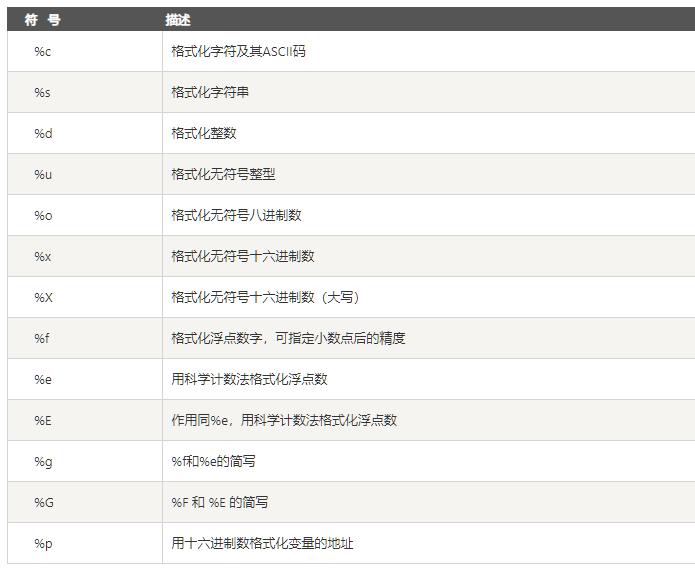# Python基础-字符串

### 字符串格式化

#### 百分号占位

tmp = '你好 我是%s，我今天挣了%.2f元钱'
value = ('小明',5.32567)
str = tmp % value
print(str)#### 模板字符串

from string import Template
tmp1 = Template('你好 我是$name，我今天挣了$num元钱')
str = tmp1.substitute(name="小红",num="6")
print(str)

#### format格式化

str = "{},{}还有{}".format("小明","小红","小强")
print(str)

str = "{2},{1}还有{4}，你们三个里面只有{1}的学习最好".format("小明","小红","小强","小芳","小黑")
print(str)

str = "今天是{name}的{age}岁生日".format(name="小明",age=18)
print(str)

#### f字符串

f字符串是python3.6新加入的一种格式化字符串的方式，对于新写的代码推荐使用这种方式来解决格式化的需求。

name = "小明"
age = 18
str = f"今天是{name}的{age}岁生日"
print(str)

### 常用方法

#### 字符串居中 center()

name = "我是小明"
print(name)
print(name.center(10))

我是小明

name = "我是小明"
print(name)
print(name.center(10,'*'))

***我是小明***

#### 查找字符串 find()

name = "我是小明"
print(name.find('小明'))

2

name = "我是小明"
print(name.find('小明',0,3))

-1

#### 大小写转换 upper、lower、title

words = "abc"
print(words.upper())

ABC

words = "ABC"
print(words.lower())

abc

words = "my name is bob"
print(words.title())

My Name Is Bob

#### 打散字符串 split()

words = "my name is bob"
print(words.split())

['my', 'name', 'is', 'bob']

words = "/usr/bin/env"
print(words.split('/'))

['', 'usr', 'bin', 'env']

#### 序列合并字符串 join()

words = ['', 'usr', 'bin', 'env']
print('/'.join(words))

/usr/bin/env

#### 去除左右空白 strip()

strip方法可以删除字符串左右两边的空白字符，但是不包含中间的空白。

words = "    my name is bob   "
print(words.strip())

my name is bob

words = "********my name is bob***"
print(words.strip('*'))

my name is bob

#### 字符串替换 replace()

words = "my name is bob, my name is bob"
print(words.replace('bob','小明'))

my name is 小明, my name is 小明

### 三引号

words = '''
<li class="dh1">
<ul class="dh2">
<li><a href="xs2.html" target="mainFrame">宇宙</a></li>
<li><a href="xs2.html" target="mainFrame">哲学</a></li>
<li><a href="xs2.html" target="mainFrame">电子商务</a></li>
<li> <a href="xs3.html" target="mainFrame">互联网</a></li>
</ul>
</li>
'''
print(words)

<li class="dh1">
<ul class="dh2">
<li><a href="xs2.html" target="mainFrame">宇宙</a></li>
<li><a href="xs2.html" target="mainFrame">哲学</a></li>
<li><a href="xs2.html" target="mainFrame">电子商务</a></li>
<li> <a href="xs3.html" target="mainFrame">互联网</a></li>
</ul>
</li>

name = ('大学','中学','小学','幼儿园')
words = '''
<li class="dh1">
<ul class="dh2">
<li><a href="xs2.html" target="mainFrame">%s</a></li>
<li><a href="xs2.html" target="mainFrame">%s</a></li>
<li><a href="xs2.html" target="mainFrame">%s</a></li>
<li> <a href="xs3.html" target="mainFrame">%s</a></li>
</ul>
</li>
'''
print(words % name)

<li class="dh1">
<ul class="dh2">
<li><a href="xs2.html" target="mainFrame">大学</a></li>
<li><a href="xs2.html" target="mainFrame">中学</a></li>
<li><a href="xs2.html" target="mainFrame">小学</a></li>
<li> <a href="xs3.html" target="mainFrame">幼儿园</a></li>
</ul>
</li>

### 判断是否满足特定条件

#### 纯字母组成 isalpha()

words = 'abc'
print(words.isalpha())

True

#### 纯数字组成 isdigit()

words = '123456'
print(words.isdigit())

True

#### 字母数字组成 isalnum()

words = 'abcd333'
print(words.isalnum())

True

words = 'abcd3 33'
print(words.isalnum())

False

#### 全大写字母 isupper()

words = ' ABCDEF123456'
print(words.isupper())

True

#### 全小写字母 islower()

words = ' abc123456'
print(words.islower())

True

### 结语

posted @ 2020-06-22 16:41  不该相遇在秋天  阅读(148)  评论(0编辑  收藏